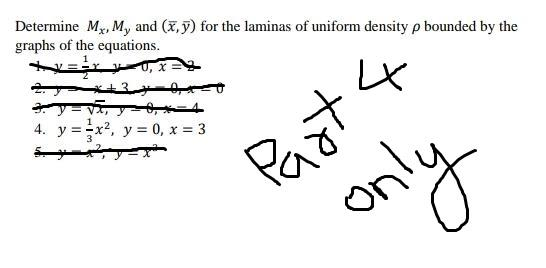### Create an Account

Already have account?

### Forgot Your Password ?

Home / Questions / Determine Mx, M, and (x,y) for the laminas of uniform density p bounded by the graphs of t...

# Determine Mx, M, and (x,y) for the laminas of uniform density p bounded by the graphs of the equations. LX Dyavi ----- 4. y ==x2, y = 0, x = 3 रो only

Determine Mx, M, and (x,y) for the laminas of uniform density p bounded by the graphs of the equations. LX Dyavi ----- 4. y ==x2, y = 0, x = 3 रो onlyApr 13 2021 View more View Less

#### Answer (Solved)Subscribe To Get Solution